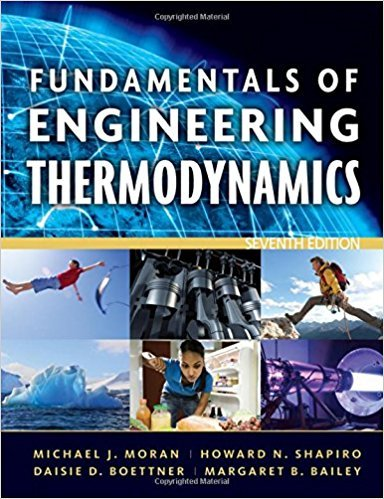×
Get Full Access to Fundamentals Of Engineering Thermodynamics - 7 Edition - Chapter 2 - Problem 1p
Get Full Access to Fundamentals Of Engineering Thermodynamics - 7 Edition - Chapter 2 - Problem 1p

×

# Exploring Energy ConceptsA baseball has a mass of 0.3 lb.ISBN: 9780470495902 50

## Solution for problem 1P Chapter 2

Fundamentals of Engineering Thermodynamics | 7th Edition

• Textbook Solutions
• 2901 Step-by-step solutions solved by professors and subject experts
• Get 24/7 help from StudySoup virtual teaching assistantsFundamentals of Engineering Thermodynamics | 7th Edition

4 5 1 364 Reviews
30
2
Problem 1P

Problem 1P

Exploring Energy Concepts

A baseball has a mass of 0.3 lb. What is the kinetic energy relative to home plate of a 94 mile per hour fastball, in Btu?Fig. P2.1

Step-by-Step Solution:

Solution

Step 1 of 2

We need to find out the kinetic energy relative to home plate of afastball.

The mass of the baseball is given asWe can convert it into SI unit,The velocity is given as,We can convert it into SI unit,Step 2 of 2

##### ISBN: 9780470495902

Unlock Textbook Solution